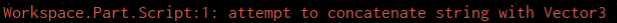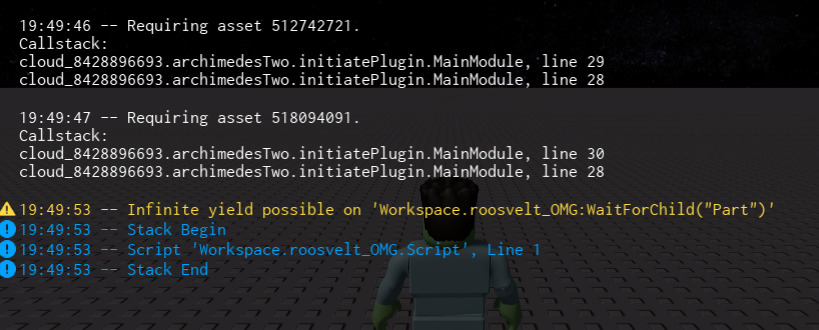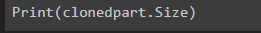# I'm trying to know the size of a ball from a script but I can't find how

Can anyone please tell me how to know the size of a ball through a script? I’ve been trying for more than 3 hours but I can’t find how

``````while true do
local clonedpart = game.Workspace.Planets:WaitForChild("ClonedPlanet")
clonedpart:GetPropertyChangedSignal("Size"):Connect(function ()
if clonedpart.Size.X >= 1800 then
clonedpart.Material = "Neon"
local randomcolor = math.random(1,3)
if randomcolor == 3 then
clonedpart.Color = Color3.new(1, 1, 0)
end
if randomcolor == 3 then
clonedpart.Color = Color3.new(1, 1, 1)
end
if randomcolor == 3 then
clonedpart.Color = Color3.new(0, 0, 0)
end
end

end)
end

``````
1 Like

All that you need to do is part.Size. This gives you the size of the part, or ball in this case. As for your code. If the number is three then it will run all three if statements and end up being `Color3.new(0,0,0)`

1 Liketry

``````Print(clonedpart.Size)
``````

and run the game and look it output

nothing happens, it just prints some code that i have there

it should print the vector3 size of the part send me a picture of what it printthats a plugin, also where did you put thiscode at?

at the botton of

``````clonedpart:GetPropertyChangedSignal("Size"):Connect(function ()
``````

is the clonedpart a basepart or other instance?

its on a folder named Planets on workspace

Oh thats weird i do not know what is wrong maybe somebody else can help

1 Like

You still access the size if the object by using `object.Size`. IDK why it is giving the concatenate error, but if you really need to print it, just use

``````print(size.X,size.Y,size.Z)
``````
1 Like

Also, why is it in a `While true do` loop? There is nothing being looped, so there is no reason to loop it at all. With the loop, the script should not work at all and the execution time will run out.

If this is running on a server script, then you do not need the `:WaitForChild()` either.

One last thing… What exactly is it that you want to achieve?

The script is being executed as a normal script, no local or module. The while true do loop makes an infinite planet spawn system and I’m trying to calculate the size of a planet to make it a sun (neon.)

The correct way to find the radius of a sphere in Roblox is by doing:

``````local function getSphereRadius(sphere)
local size = sphere.Size
return math.min(size.x, size.y, size.z)/2
end
``````

Best regards,
Octonions

I think you meant `math.min` because if you use max then when one side is smaller, the sphere will be smaller, and not reach the full size. Spheres take on a radius of half of the smallest size in roblox, not the largest.

Yes. Thanks for letting me know. I have fixed it.

This topic was automatically closed 14 days after the last reply. New replies are no longer allowed.